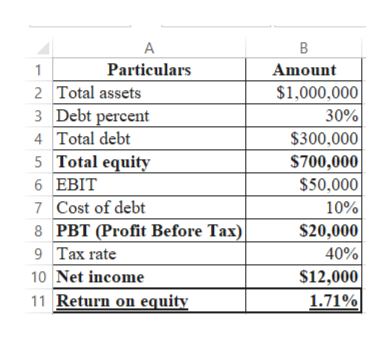# A firm has total assets of \$1,000,000 and a debt ratio of 30 percent. Currently, it has sales of \$2,500,000, total fixed costs of \$1,000,000, and EBIT of \$50,000.  If the firm's before-tax cost of debt is 10 percent and the firm's tax rate is 40 percent, what is the firm's ROE?

Question
6 views

A firm has total assets of \$1,000,000 and a debt ratio of 30 percent. Currently, it has sales of \$2,500,000, total fixed costs of \$1,000,000, and EBIT of \$50,000.  If the firm's before-tax cost of debt is 10 percent and the firm's tax rate is 40 percent, what is the firm's ROE?

check_circle

star
star
star
star
star
1 Rating
Step 1

Return on equity (ROE):

It is a profitability measure that is related with the firm's equity. It is used mainly to compare the firm's performance within the same industry. ROE indicates the efficient usage of investment by the firm to generate growth in the earnings.

Step 2

Computation of Return on Equity (ROE):

Hence, the firm's ROE is 1.71%...help_outlineImage TranscriptioncloseParticulars 2 Total assets 3 Debt percent 4 Total debt Amount \$1,000,000 30% \$300,000 \$700,000 1 5 Total equity 6 EBIT \$50,000 10% \$20,000 7 Cost of debt 8 PBT (Profit Before Tax) 9 Tax rate 40% \$12,000 1.71% 10 Net income 11 Return on equity fullscreen

### Want to see the full answer?

See Solution

#### Want to see this answer and more?

Solutions are written by subject experts who are available 24/7. Questions are typically answered within 1 hour.*

See Solution
*Response times may vary by subject and question.
Tagged in